# 使用 COMSOL 对半导体器件材料的能带结构进行仿真

2020年 12月 1日

### 哈密顿矩阵

(1)

\sum_{n=1}^N \mathbf{H}_{mn} \psi_{n}(\mathbf{r},t) = -i \hbar \frac{\partial}{\partial t}\psi_{m}(\mathbf{r},t) \, , \qquad m = 1, 2, 3, \,\dots\, N

(2)

\mathbf{H}_{mn} = \,\,\frac{+\hbar^2}{2\,m_e}\sum_{ i,j\in {1,2,3}} \left[\frac{i\, \partial}{\partial r_i} \,A_{ij}^{mn}(\mathbf{r}) \, \frac{i\, \partial}{\partial r_j}+ \frac{i\, \partial}{\partial r_i}\,H_i^{mn(1R)}(\mathbf{r})+ H_j^{mn(1L)}(\mathbf{r}) \, \frac{i\, \partial}
{\partial r_j}+ H^{mn(0)}(\mathbf{r})\right]

### 模型系统

(3)

\mathbf{H}^U=\left[\begin{array}{ccc}
F & K_t & -i H_t \\
K_t & G & \Delta-i H_t \\
i H_t & \Delta+i H_t & \lambda \\
\end{array}\right]

(4)

F=\Delta_1+\Delta_2+\lambda+\theta

(5)

\lambda=\frac{\hbar^2}
{2m_e}\left[A_1 k_z^2+A_2(k_x^2+k_y^2)\right]+\lambda_\epsilon

(6)

\theta=\frac{\hbar^2}{2m_e}
\left[A_3 k_z^2+A_4(k_x^2+k_y^2)\right]+\theta_\epsilon

(7)

\lambda=\frac{\hbar^2}{2m_e}\left[
i\frac{\partial}{\partial z} A_1 i\frac{\partial}{\partial z}
+ i\frac{\partial}{\partial x} A_2 i\frac{\partial}{\partial x}
\right]+\lambda_\epsilon

(8)

\theta=\frac{\hbar^2}{2m_e}\left[i\frac{\partial}
{\partial z} A_3 i\frac{\partial}{\partial z}+ i\frac{\partial}
{\partial x} A_4 i\frac{\partial}{\partial x}\right]+\theta_\epsilon

(9)

\lambda_\epsilon = D_1 \epsilon_{zz} + D_2(\epsilon_{xx}+\epsilon_{yy})

(10)

\theta_\epsilon = D_3 \epsilon_{zz} + D_4(\epsilon_{xx}+\epsilon_{yy})

(11)

F=\frac{\hbar^2}{2m_e}
\left[i\frac{\partial}{\partial z} (A_1+A_3) i\frac{\partial}{\partial z}
+ i\frac{\partial}{\partial x} (A_2+A_4) i\frac{\partial}{\partial x}
\right]\Delta_1\Delta_2+\lambda_\epsilon+\theta_\epsilon

### COMSOL Multiphysics® 模型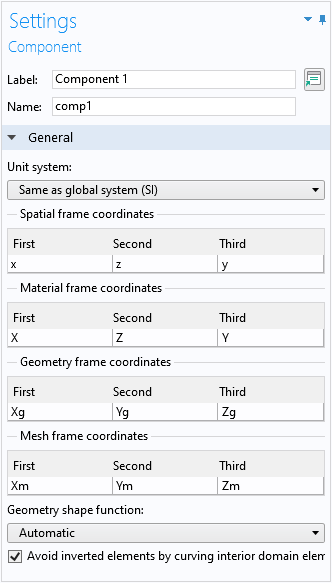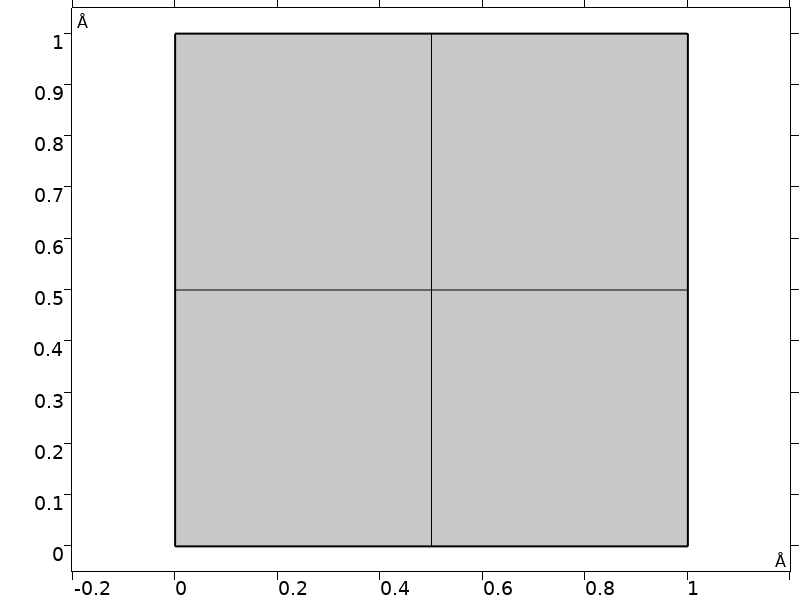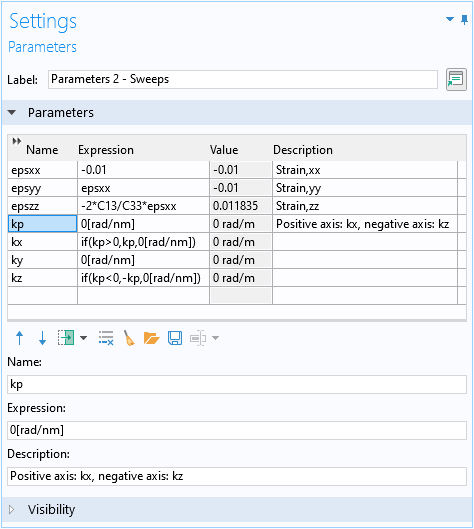kxkz 的双轴一维绘图设置参数  kp

### 解析解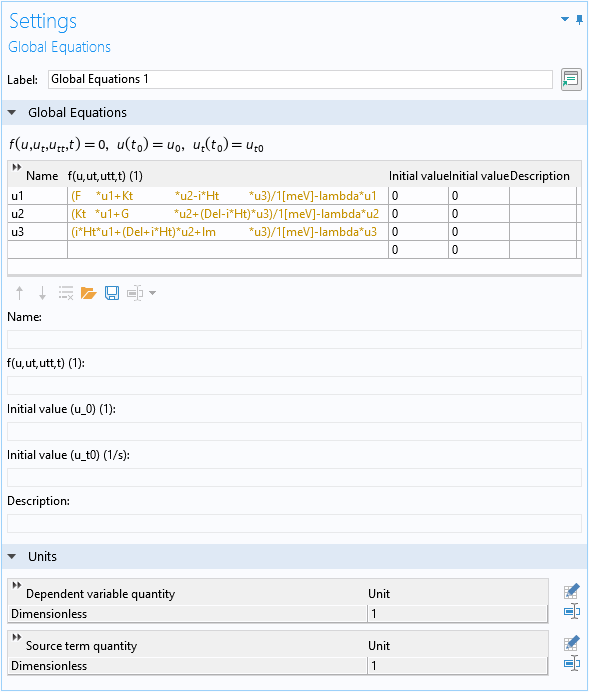### 求解薛定谔方程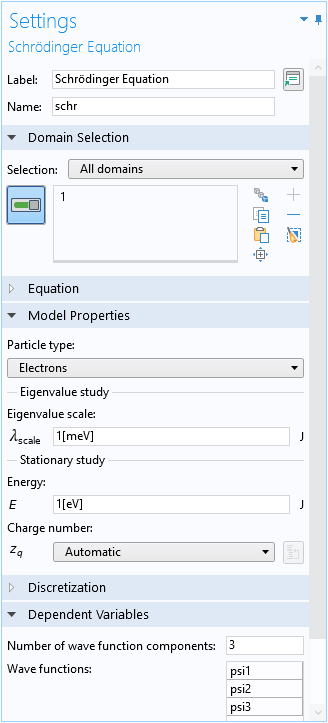### 哈密顿矩阵输入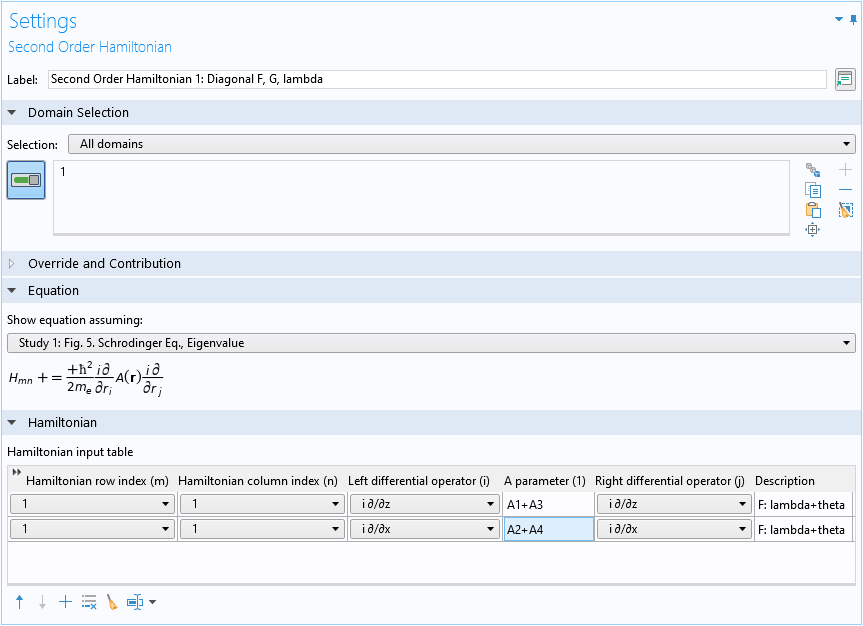#### 复制/粘贴表格行

(12)

G=\Delta_1-\Delta_2+\lambda+\theta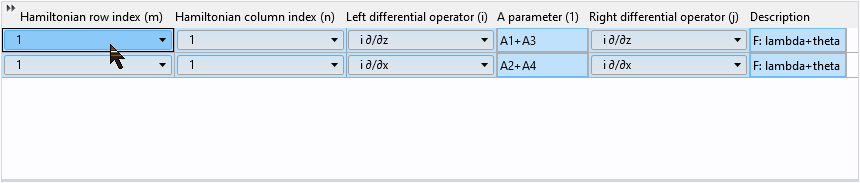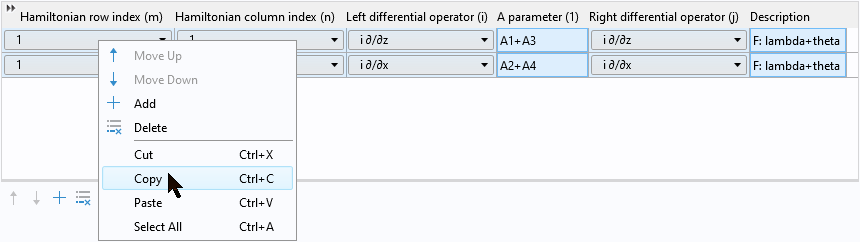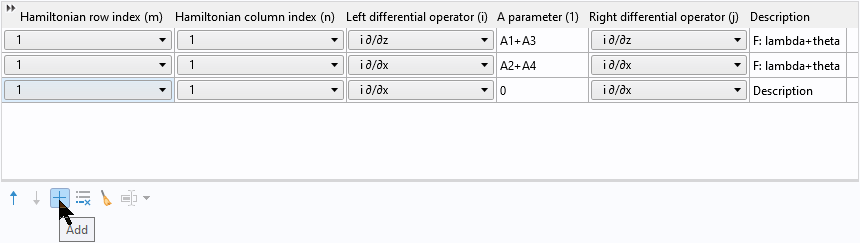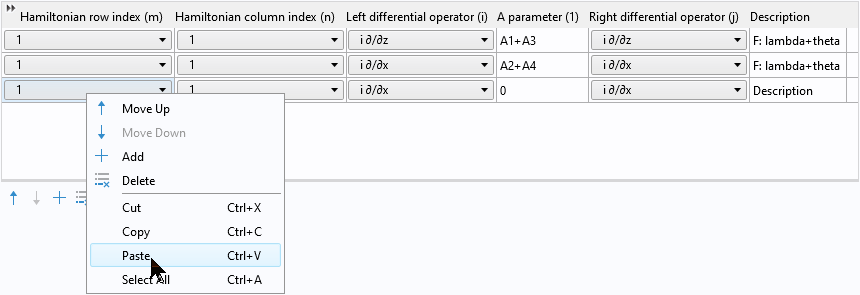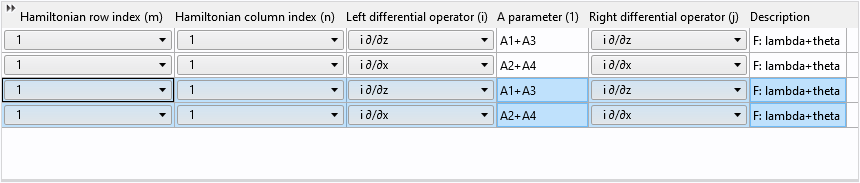### 禁用默认有效质量贡献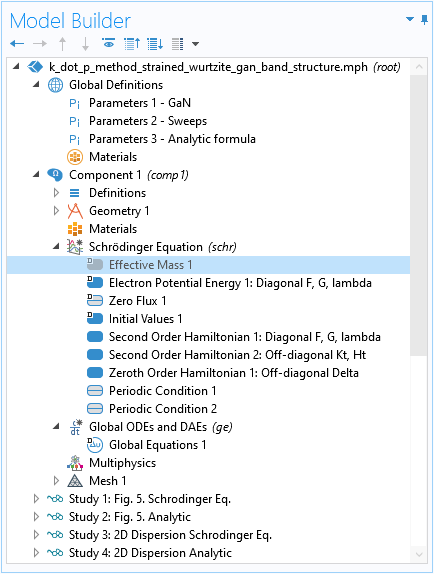### 没有算子的项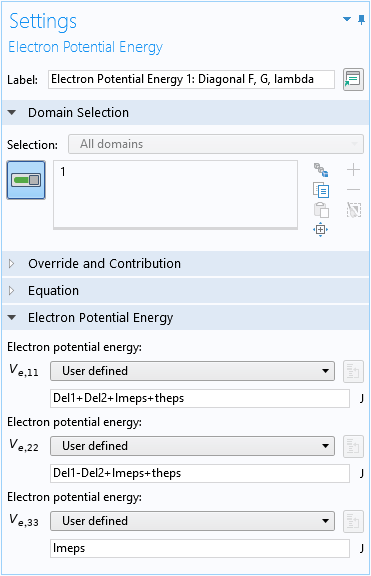### 非对角元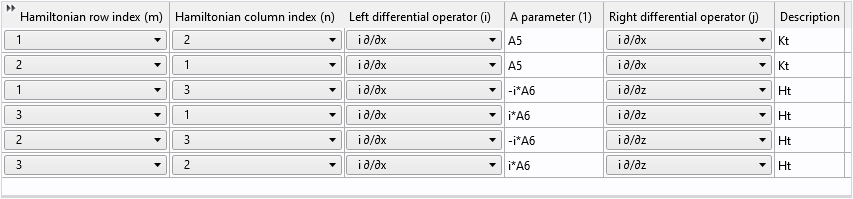### 其他设置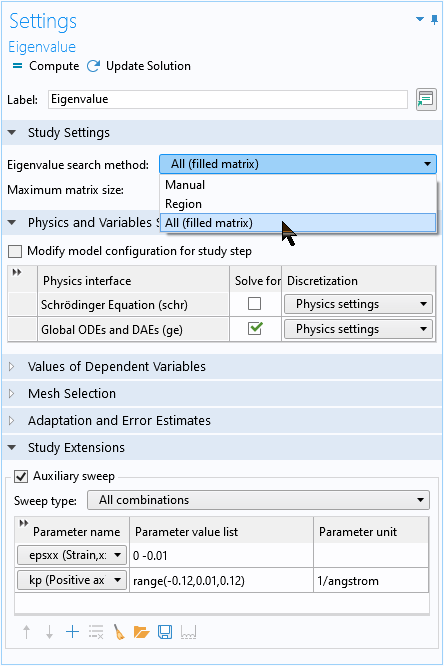### 未发生应变和发生应变的纤锌矿晶体的已计算能带结构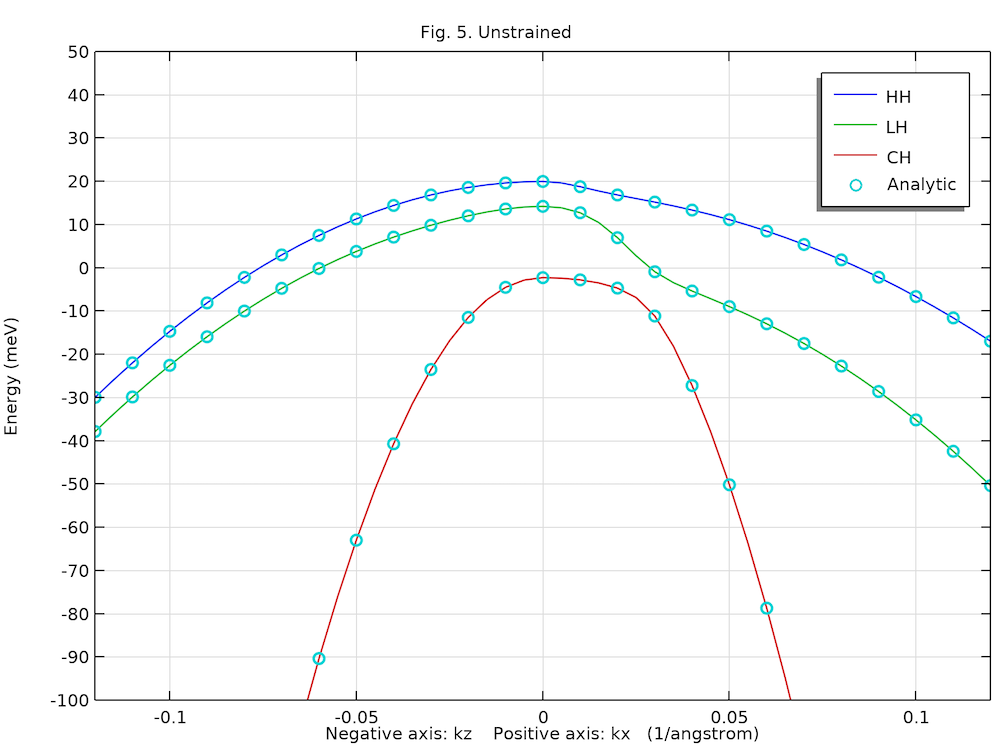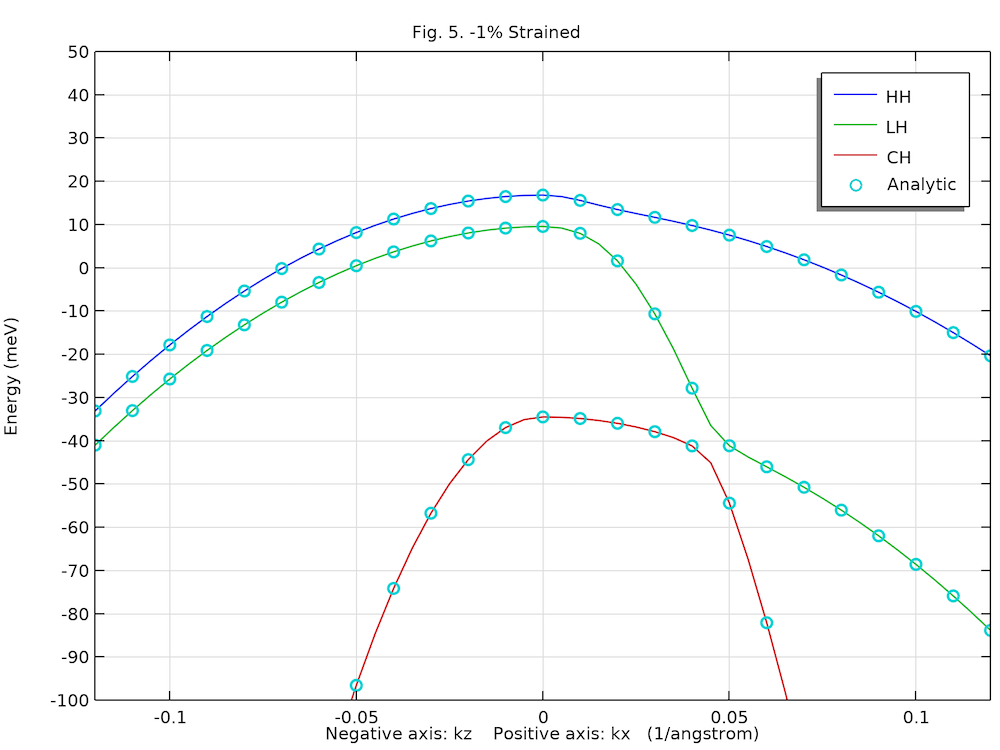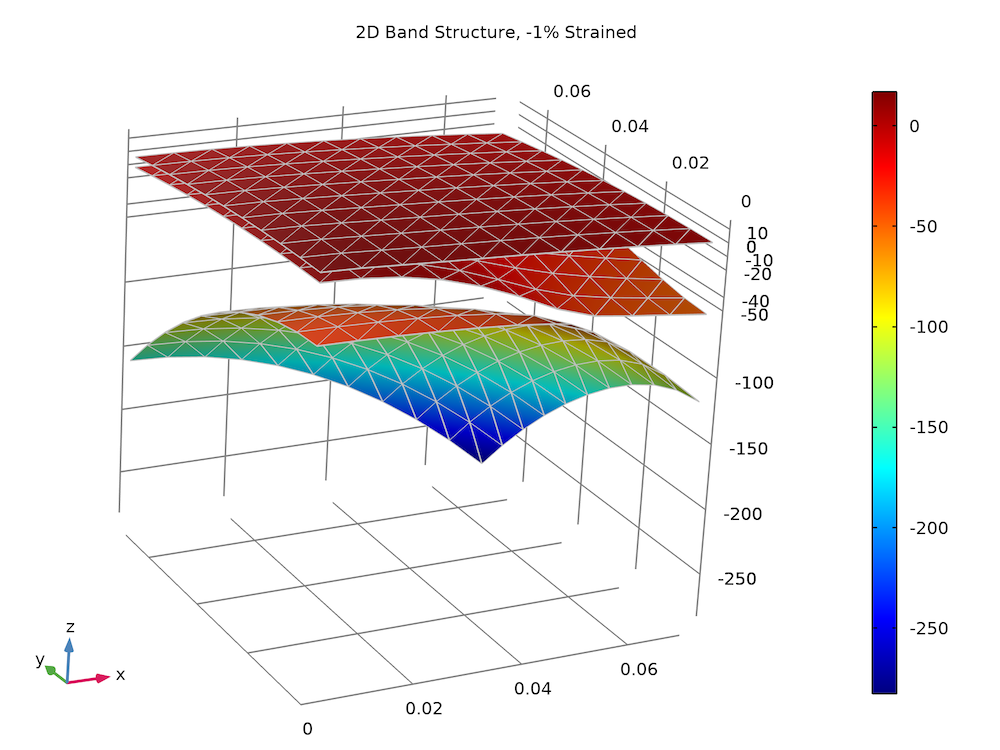### 动手尝试

1. L. Chuang and C.S. Chang, “k·p method for strained wurtzite semiconductors,” Phys. Rev. B, vol. 54, p. 2491, 1996.